# Introducing Runsums - a sum of consecutive integers

This page investigates numbers that are the sum of a run of whole numbers, such as 5+6+7 or 2+3+4+5+6, their properties and fascinating patterns.
The calculators on this page require JavaScript but you appear to have switched JavaScript off (it is disabled). Please go to the Preferences for this browser and enable it if you want to use the calculators, then Reload this page.
The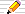icon means there is a You do the maths... section of questions to start your own investigations. The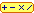calculator icon indicates that there is a live interactive calculator in that section.

## Runsums: Sums of Runs

A run of numbers is a sequence of consecutive whole numbers i.e. with no gaps in the sequence,
 such as 2, 3, 4; and 7 is a run even though it contains just a single number but not 5, 6, 8, 9 because 7 is missing and not 2, 3, 3 because 3 is repeated
Every number is therefore a sum of numbers in a run because we can have a run with just a single number in it!
Many numbers can also be written as a sum of a run of 2 or more numbers. For example:
 9 = 2 + 3 + 4 = 4 + 5 10 = 1 + 2 + 3 + 4 11 = 5 + 6 12 = 3 + 4 + 5 13 = 6 + 7 14 = 2 + 3 + 4 + 5
For convenience, the sum of a run of numbers we will call a runsum.

### Making a table of runsums

Since every runsum is determined by its staring and ending number, we can make a table of the sums of integers between the two values:
SUMS 1 2 3 4 5 6 7 8 To From 1 1+2=3 1+2+3=6 1+2+3+4=10 1+2+3+4+5=15 21 28 36 2 2+3=5 2+3+4=9 2+3+4+5=14 20 27 35 3 3+4=7 3+4+5=12 18 25 33 4 4+5=9 15 22 30 5 11 18 26 6 13 21 7 15 8 9
Each entry in the table is the sum of the whole numbers starting at the from value on the left of its row and ending at the to value at the top of its column. A few entries are shown in detail.
So first fill in the rest of the entries in the table and then answer these questions:

#### You do the maths...

1. 9 occurs in row 2 and column 4 and so the sum of the numbers from 2 to 4 is 9: checking: 2+3+4 is 9.
So by looking at the numbers in the table, we can find runsums.
Find two more locations in the table with 9 in it. What runsums are they?
2. How many runsums can you find for 12?
3. How many runsums for 15 can you find in the table?
4. Use your table to find all the runsums of 2, 3, 4, 5, 6, 7, 8, 9 and 10.
There is only one runsum for 1 and for 2. If there is more than one runsum for a number, write each runsum on a line of its own in the right box.
Put your results into a new table like this:
 n runsumsof n 1 2 3 4 5 6 7 8 9 ... 1 2 31+2 4 ? ? ? ? 92+3+44+5 ...
5. Is our table big enough to find all the runsums for 20?
No, because some of the lower rows do not go far enough to the right. Extend your table so that you are sure all the numbers up to 20 are in your extended table and then answer these questions:
1. Find all the runsums of 15
2. Extend your new table of all runsums so that it includes all the runsums up to 20.

## Triangular Numbers

By placing coins (cans, boxes) in rows, one row above another, we can make triangular patterns: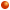...

In these triangles, there are 1, 3, 6 and 10 balls.
How many will there be in the next triangle, the one with 5 on the bottom row?

The numbers in this series are called Triangular Numbers and we give them the names T(1), T(2), T(3) and so on e.g.T(1) = 1, T(2) = 3, T(3) = 6. The tenth Triangular number's pattern will have 10 items on the bottom row and 1 on the top row, a total number of T(10) objects in it.

Looking at all the rows in one pattern above, the total number of boxes is:
1 = 1 in the first
1 + 2 = 3 in the second
1 + 2 + 3 = 6 in the third
1 + 2 + 3 + 4 = 10 in the fourth
and so on.
So can you now calculate T(10) without drawing it?
T(10) = 1 + 2 + 3 + 4 + 5 + 6 + 7 + 8 + 9 + 10 = 55.

To summarise:

All triangular numbers are runsums - the special runsums that begin at 1.
T(n)'s longest row is of length n.
T(n) is the sum of the numbers from 1 up to and including n.

The first 12 Triangular numbers
 i T(i) 0 1 2 3 4 5 6 7 8 9 10 11 12 0 1 3 6 10 15 21 28 36 45 55 66 78

### Runsums and Triangular Numbers

If we look at a triangular pattern above and take off a triangle from the top of it, we will still be left with a runsum, but the smallest row, the topmost one, will be bigger than 1. Here we take off T(3)=6 shown in green from T(6)=21: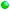This shows that T(6) - T(3) = 21 - 6 =15. Looking at the red rows only we see 15 can be written as 4 + 5 + 6, a runsum.

This will work for any runsum. For instance, 11 = 5 + 6. The largest number in the runsum is 6 so we start with T(6)'s triangle. But our runsum begins at 5, so we have removed T(4) = 1 + 2 + 3 + 4. Therefore

11 = 5 + 6 = T(6) - T(4)

Any runsum can be written as the difference between two triangular numbers
If N = a + (a+1) + ... + b then N = T(b) – T(a–1)

Note that a can be 1 so that N = T(b) –T(0) = T(b) and we include the triangular numbers themselves as runsums.

The shape of a triangle with its top cut off is called a trapezium and so runsums are also called trapezoidal arrangements or trapezoids (in article 1999.1.6 in the Journal of Integer Sequences, Vol 2 (1999) by Tom Verhoeff of Eindhoven University of Technology).

## Numbers with several runsums

By looking at all the possibilities, we find that 4 cannot be written as a runsum of more than a single number.
Every number has a runsum consisting of that number alone, so such runsums are "trivial" and we want to find "interesting" runsums!
Is 4 perhaps just a special case? Are there other numbers that have no "interesting" runsums? How can we tell if a number has an "interesting" runsum or not?

### Trivial and interesting runsums

#### You do the maths...

1. Find another number that has no single runsums (hint: apart from 4, there is another one less than 10).
2. What is the SMALLEST number that is a sum of a run of three numbers?
3. What is the smallest sum of four numbers in a run?
4. .. and five?
5. By spotting the pattern can you tell me what is the smallest number which is a sum of one hundred consecutive numbers - but I want to know HOW you know too!
6. Mathematicians call the single-number runsums trivial (meaning that they are not very interesting cases) and the others are non-trivial or proper runsums (the interesting ones).
I went on looking for a proper runsum for 4 and 8, and I still couldn't find any.
I did notice that 9 was also interesting because it was the first number I that had three different runsums:
9 = 2 + 3 + 4 = 4 + 5

I then found that 27 had four different runsums, what were they?
7. What is the smallest number with four different runsums?
8. I thought I'd see if I could find a number with five runsums but when I was searching, I found a number with six runsums!
What is the smallest six runsum number that I could have found?
9. How many numbers between 2 and 100 have six different runsums?
10. While trying to answer the last question, I did find just one number with exactly five runsums. What was it?
11. There were still some numbers in the range 2 to 100 that seemed to have no runsums longer than one number.
Can you spot a pattern in the list of such numbers?
12. What is special about the numbers with exactly three runsums (such as 9)?

## A Runsum Calculator

Here is an online calculator that can help with your investigations.
All numbers have a (trivial) runsum of length 1, namely the number itself.
Runsums are abbreviated to the first and last numbers in the run, for example:
3 + 4 + 5 is shown as 3..5 by the Calculator.
C A L C U L A T O R
Italic parts are optional.
the sum of all the whole numbers from up to
 all shortest longest runs with a sum of up to
 with sum starting ending at runs
 runs of length
 from to that are the sums of exactly at least up to runs
 with runsums
 T() up to T()
R E S U L T S: Runsums Friend & Neighbours
It will instantly tell you that
• the sum of the numbers from 200 to 800 is 300500;
• there are 12 runsums for 9999 and it will show you what they are.
Here are some more investigations to do using the Runsum Calculator:

### You do the maths...

1. What is the sum of all the numbers from 2 to 8?
2. from 20 to 80?
3. from 200 to 800? [Why is it not 10 times the answer above?]
4. Can you spot the pattern in these answers?
1. Try the same thing but for 2 to 9, 20 to 90, etc. Is there a pattern now?
2. What about 3 to 7, 30 to 70, and so on? Is there a pattern here?
3. Try 10 to 14, 110 to 114, 1110 to 1114 and so on. How would you describe this pattern?
4. Can you find more number patterns like these?
Please email me (address at foot of page) with your answers to these questions and the results will be put on this site for others to see. There are probably many kinds of patterns here!
5. A more advanced Project (age 15 and above):
Let's use the notation sum(a,b) to mean the sum of all the numbers from a to b.
So sum(2,5) = 2+3+4+5 = 14. What is
1. sum(1,2)?
2. sum(1,3)?
3. sum(1,4)?
4. Find a formula for sum(1,b).
5. What is the name for the series of numbers of the previous question?
1. What is sum(10,20)?
2. Suppose we know that sum(1,20) = 210 and sum(1,9) = 45, how can we use these two values to compute sum(10,20)?
3. Using your formula for sum(1,b), use it to write down a formula for sum(a,b).

Here is my page of Runsum Facts and Figures which provides answers to many of the questions above.

## Quick Ways To Calculate Runsums

First we find a formula for any runsum between two given numbers that is easy to remember and then we adapt it to find an easier way to use it for computing runsums in your head without a calculator.

### A Basic Formula and proof

If we want to sum the numbers from 10 to 20, we can arrange two copies of the numbers like this, the top one listing them forwards form 10 to 20 and the bottom line with the numbers listed backwards from 20 down to 10:
 10 11 12 13 14 15 16 17 18 19 20 20 19 18 17 16 15 14 13 12 11 10
Note that each column adds up to the same value: 30.
The first column is also just the first value plus the last.
How many columns are there? From 10 to 20 is 20-10 plus one = 11
Thus the sum of all the numbers in the table is 11 copies of 30 or 330.

This adds two copies of the list so the list 10 to 20 has a sum of 330/2 = 165.

This method applies to all lists of consecutive numbers from a to b.
Each column has the same sum: a+b and there are b–a+1 columns.
So (b–a+1)(a+b) is twice the sum of the numbers from a to b:
Let's use sum(a,b) to mean a + (a+1) + (a+2) + ... + b
So our first forumula is

 sum(a,b) = (b – a + 1)(a + b) = (b – a + 1) (a + b) 2 2
The second form gives us an easy way to remember this formula:
sum(a,b) = number of values × average value
where the average value is (a + b)/2 and
the number of values is b – a + 1
This formula is fairly easy to remember but not so good to use to calculate runsums in your head:
Here is another example : What is the sum of the numbers from 6 to 20?
There are (20 – 6 + 1) = 15 columns each with a sum of 6 + 20 = 26. So twice the sum we want is 15 × 26. So the sum of the numbers from 6 to 20 is half of this: 15 × 13 (hmmm --- out with the calculator at this point perhaps!) which turns out to be 195.

When the runsums start at 1 we have a formula for the sum of the first n numbers, or the n-th triangle number T(n):

 T(n) = 1 + 2 + ... + n = n (n + 1) 2

### An easier formula for mental arithmetic

Rearranging our formula above we have:
sum(a,b) =
 (b – a + 1)(a + b)2
=
 b2 – a2 + b + a2
or sum(a,b) is (the difference of the squares of a and a PLUS their sum) over 2
So, for sum(6,20) we have 202 = 400 and 62 36, with a difference of 364.
The sum of 6 + 20 = 26 which we add on to 364 to get 390. Halving this we have 195 as before.
These sums are make excellent practice for your mental arithmetic skills!!

## Friends and Neighbours

Where a number (sum) has more than one runsum, we can find more patterns. In this section we look for
runsums that have one number in common
such as 2 + 3 + 4 = 4 + 5.
Since the runsums have something in common, we will call them friends.
Other pairs of runsums (for the same sum) are neighbours if one starts with the value next to where the other ends, such as in 4 + 5 + 6 = 7 + 8.

### Friendly runsums

We are calling the two runsums 2 + 3 + 4 and 4 + 5 friends since they have the same sum and share a number in common (4), so that one runsum starts where the other ends.
Are there any more?
21 is the sum for the next pair of friends: 1 + 2 + 3 + 4 + 5 + 6 = 6 + 7 + 8 .
There are quite a lot of friendly runsums when you start to look.
The sums with the pair of friendly runsums here are 9 and 21 and the series of such sums is:
 9= 2+3+4 = 4+5 21= 1+2+3+4+5+6 = 6+7+8 30= 6+7+8+9 = 9+10+11 42= 3+4+5+6+7+8+9 = 9+10+11+12 65= 2+3+4+5+6+7+8+9+10+11 = 11+12+13+14+15 70= 12+13+14+15+16 = 16+17+18+19
and continues with 99, 105, 117, 133, 135, 154, 175, 180, ... see A110701
The common numbers in these friendly runsums are 4,6,9,11,14,15,16 and the series continues indefinitely (see Sloane's A094550)

If we omit the common number, the new series of sums is:
5,15,21,33,54,54,85,90,100, ... (see Sloane's A110702)

### Balancing Numbers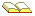A Behera and G K Panda in a paper entitled On The Square Roots of Triangular Numbers in Fibonacci Quarterly, 1999, pages 98-105, use the term balancing number for the number common to two Friendly runsums where both of the following conditions must hold:
1. both runsums consist of more than one number
2. one of the two runsums begins at 1
We have 2 + 3 + 4 = 4 + 5 but 4 (the common number) is not a balancer since neither of its runsums begins with 1.
However, 6 is a balancing number since
1 + 2 + 3 + 4 + 5 + 6 = 6 + 7 + 8
They call the number of terms in the runsum commencing n + 1 the balancer. Thus for the balancing number 6, we have the balancer 2 because
1 + 2 + 3 + 4 + 5 + 6 = 6 + (6+1) + (6+2)
Behera and Panda show that there is a connection between the balancing number n and a balancer r as follows:
 n2 = (n + r)(n + r + 1)2
 r = √(8 n2+1) – 2n – 12
and so n2 must be a Triangular number and 8 n2 + 1 must be a perfect square.
They also show that in the sequence of balancing numbers, each is 6 times the previous number minus the number before that:
 B(0) = 1 B(1) = 6 B(2) = 35 B(i) = 6 B(i–1) – B(i–2) for i > 2
This is A001109: 1, 6, 35, 204, 1189, 6930, 40391, 235416, ....

In the same way that the Fibonacci Numbers have a Binet Formula which explicitly gives Fib(n) in terms of n, the balancing numbers have the formula:

 B(n) = Gn+1 – gn+1 where G = 3 + √8, g = 3 – √8G – g
In the same way that the ratio of Fibonacci numbers tends to Phi, the ratio of balancing numbers tends to G which is also 1/g and it has the periodic continued fraction expansion of [ 5; 1,4] = [6; –6,6]:-
 6 + 1 −6 + 1 6 + 1 −6 + 1 ...K Liptai in Fibonacci Balancing Numbers, Fibonacci Quarterly,2004, pages 330-340, showed that 1 is the only Fibonacci number that is a balancing number too.

### Neighbourly Runsums

Here are two runsums of 15: 4 + 5 + 6 = 7 + 8. Notice that second continues the run started in the first and also that the two runs have the same sum. So instead of two runsums (of the same sum) sharing a common value as in Friends above, we now find runsums that fit together as neighbours: as one runsum ends, the other continues without a break. We call such runsums neighbours since they are runs that are next to each other.
27 has two neighbouring runsums:
2 + 3 + 4 + 5 + 6 + 7 = 8 + 9 + 10 = 27

The ordered sequence of sums with two neighbouring runsums is:

15 = 4+5+6 = 7+8
27 = 2+3+4+5+6+7 = 8+9+10
30 = 4+5+6+7+8 = 9+10+11
42 = 9+10+11+12 = 13+14+15
75 = 3+4+5+6+7+8+9+10+11+12 = 13+14+15+16+17
90 = 16+17+18+19+20 = 21+22+23+24
and continues with 105,135,147,165 ... (see Sloane's A110703)

### A Friends and Neighbours Runsum Calculator

C A L C U L A T O R
Italic indicates an optional input
 a..b=b..c a..b=(b+1)..c
with a =
up to
and sum
up to
 a..b=b..c a..b=(b+1)..c with b = up to
R E S U L T S: Runsums Friend & Neighbours

## Polyomino Runsums

Livio Zucca has a great album of photos on Facebook where he uses a connected shape of n squares for each number n in a runsum and then arranges them into a rectangle or a square to illustrate the runsum.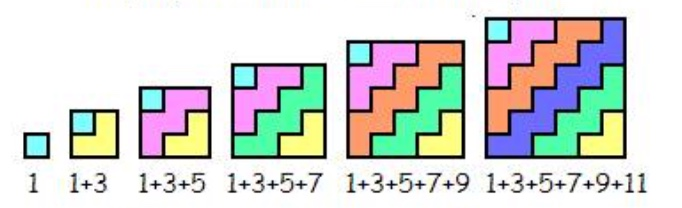For instance, let's make each odd number into a zigzag shape of squares and then we can use the fact that the sum of the first n odd numbers is n2 to make an n×n shape jigsaw:
1 + (1 + 2 ) = 1 + 3 = 4 = 22
(1 + 2) + (1 + 2 + 3 ) = 1 + 3 + 5 = 9 = 32
(1 + 2 + 3) + (1 + 2 + 3 + 4 ) = 1 + 3 + 5 + 7 = 16 = 42 ...
Because the runsums starting at 1 are the triangle numbers T(n), we have the formula
 T(n) = 1 + 2 + ... + n = n (n + 1) 2
.
This means we can make each triangle number 1 + 2 + ... + n into a rectangle too! Why?
Because one of n or n+1 is even, or, equivalently :
if n is even then T(n) can make the rectangle (n/2) ×(n+1);
if n is odd, then (n+1) is even so n × (n+1)/2 are the sides of the rectangle.
Livio also uses this and uses the same zigzag shapes for each number from 1 to n to form a rectangle with the first n numbers: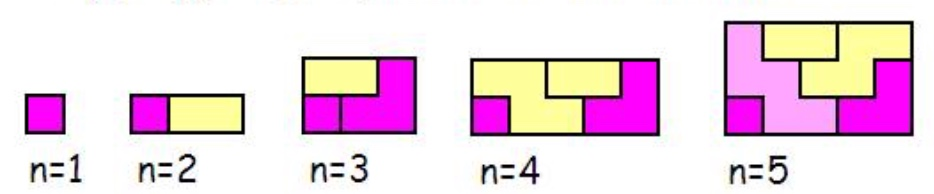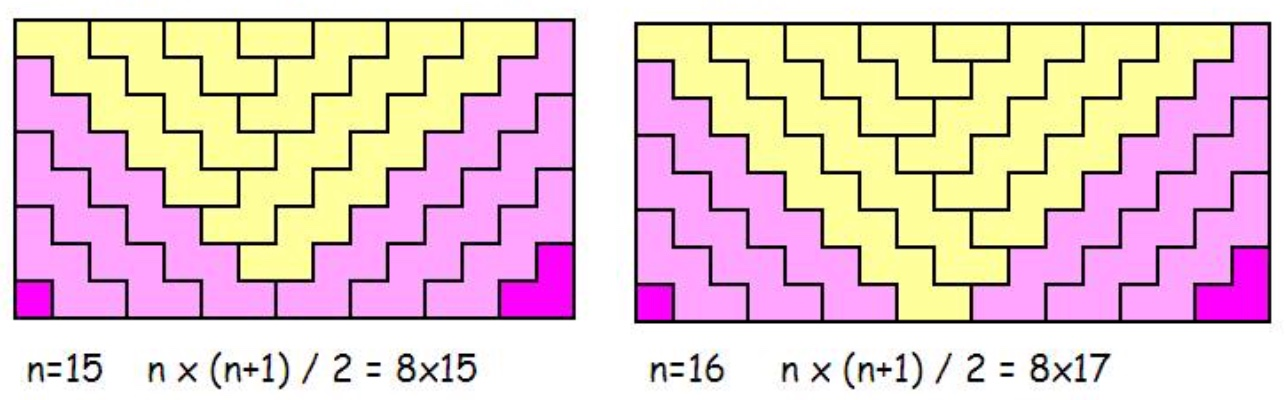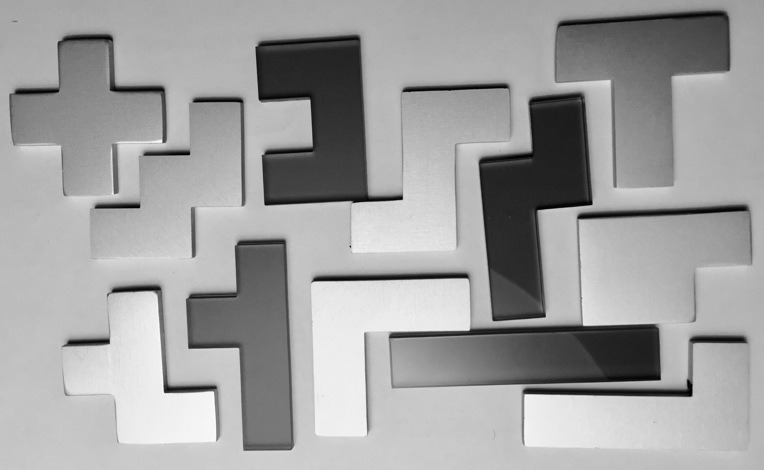Since two connected squares are a domino, any shape of n connected squares is called a polyomino with the plural polyominoes.
We can make many shapes with n connected squares and there are many puzzles, problems and games involving them.

For instance, the 12 shapes made from 5 connected squares are the 12 pentominoes shown here on the right. A polyomino shape may be rotated or turned over (making its mirror image) but they are all the same polyomino. Since the 12 shapes each have 5 squares making a total of 60 squares, we can try to arrange these into a 6×10 rectangle or a 5×12 rectangle or a 3×20 rectangle. There are many ways to solve each of these three puzzles and if you make cut out the 12 shapes it makes a nice challenge to see how long it takes you to find one solution for each of these.

The square runsums of length more than 1 are
32 52 62 72 92 = 9 has 3 runsums 2..4 = 4..5 = 9 = 25 has 3 runsums 3..7 = 12..13 = 25 = 36 has 3 runsums 1..8 = 11..13 = 36 = 49 has 3 runsums 4..10 = 24..25 = 49 = 81 has 5 runsums 5..13 = 11..16 = 26..28 = 40..41 = 81 = 100 has 3 runsums 9..16 = 18..22 = 100 = 121 has 3 runsums 6..16 = 60..61 = 121 = 144 has 3 runsums 12..20 = 47..49 = 144 = 169 has 3 runsums 7..19 = 84..85 = 169 = 196 has 3 runsums 21..28 = 25..31 = 196 = 225 has 9 runsums 4..21 = 8..22 = 18..27 = 21..29 = 35..40 = 43..47 = 74..76 = 112..113 = 225

What shapes can you use to make these into polyomino runsum jigsaw puzzles?
There is much more about making shapes out of numbers, not just connected squares, on the Polygonal and Figurate Numbers page on this site.
Now have a look at the next page on Runsums
© 1999-2018 Dr Ron Knott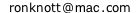created in 1999; last updated on 29 January 2018